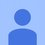# What is "Molar" Volume?

I had recently been studying about Molar Volume in chemistry. What is it? And is it okay to say that:
Density (ρ) = Molar Mass/Molar VolumeNote by Vasu Khera
2 months, 3 weeks ago

This discussion board is a place to discuss our Daily Challenges and the math and science related to those challenges. Explanations are more than just a solution — they should explain the steps and thinking strategies that you used to obtain the solution. Comments should further the discussion of math and science.

When posting on Brilliant:

• Use the emojis to react to an explanation, whether you're congratulating a job well done , or just really confused .
• Ask specific questions about the challenge or the steps in somebody's explanation. Well-posed questions can add a lot to the discussion, but posting "I don't understand!" doesn't help anyone.
• Try to contribute something new to the discussion, whether it is an extension, generalization or other idea related to the challenge.

MarkdownAppears as
*italics* or _italics_ italics
**bold** or __bold__ bold
- bulleted- list
• bulleted
• list
1. numbered2. list
1. numbered
2. list
Note: you must add a full line of space before and after lists for them to show up correctly
paragraph 1paragraph 2

paragraph 1

paragraph 2

[example link](https://brilliant.org)example link
> This is a quote
This is a quote
    # I indented these lines
# 4 spaces, and now they show
# up as a code block.

print "hello world"
# I indented these lines
# 4 spaces, and now they show
# up as a code block.

print "hello world"
MathAppears as
Remember to wrap math in $$ ... $$ or $ ... $ to ensure proper formatting.
2 \times 3 $2 \times 3$
2^{34} $2^{34}$
a_{i-1} $a_{i-1}$
\frac{2}{3} $\frac{2}{3}$
\sqrt{2} $\sqrt{2}$
\sum_{i=1}^3 $\sum_{i=1}^3$
\sin \theta $\sin \theta$
\boxed{123} $\boxed{123}$

Sort by:

- 2 months, 3 weeks ago

I thank you, buddy.

- 2 months, 3 weeks ago

No prob, chemistry is the one thing I'm good at LOL :)

- 2 months, 3 weeks ago

Can you make it more clear? Please elaborate.

- 2 months, 3 weeks ago

What should I explain? The variables?

- 2 months, 3 weeks ago

No. I mean the concept. I'm pretty confused about it.

- 2 months, 3 weeks ago

Moles is amount of matter. Molar Volume is how much volume that Mole(matter) occupies. So if a ball with mass 1 Mole occupies $1m^{3}$ volume, then the Molar Volume is $\dfrac{1m^{3}}{1 \ mole}$ or $1m^{3} \ per \ mole$

- 2 months, 3 weeks ago

But ain't "Mole" a number? How can something have a mass equal to a Mole?

- 2 months, 3 weeks ago

A mole of something has 6.022 × 10²³ atoms, so the ball has 6.022 × 10²³ number of atoms we can say. 6.022 × 10²³ is Avogadro's Number :)

- 2 months, 3 weeks ago

- 2 months, 3 weeks ago

Not the constant. The "concept", the idea, what is it about?

- 2 months, 3 weeks ago

$n=\cfrac{m}{M},m=\rho V\implies n=\cfrac{\rho V}{M}$, but $\rho=\cfrac{M}{V_m}\therefore n=\cfrac{V}{V_m}$

- 2 months, 3 weeks ago

This? (some text)

- 2 months, 3 weeks ago

No problem buddy. Don't bother.

- 2 months, 3 weeks ago

$V_m=\cfrac{V}{n}$. This is a constant with a given pressure and temperature for any gases. Standard pressure and $0^{\circ}C:22,41\text{dm}^3\cdot\text{mol}^{-1}$

- 2 months, 3 weeks ago

Yes your statement is true. Molar Volume is the amount of space that the molar mass(amount of matter) occupies. This is what gives us formula for density @Vasu Khera :)

- 2 months, 3 weeks ago